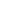eForm FDF ExampleThis example shows how to extract Unicode annotation values from an eForm FDF file.Src

 First we create an ABCpdf Doc object and read in our FDF file. [C#] ```Doc theFDF = new Doc(); theFDF.Read(Server.MapPath("../Rez/form.fdf"));``` [Visual Basic] ```Dim theFDF As Doc = New Doc() theFDF.Read(Server.MapPath("../Rez/form.fdf"))```Dest

 We find out how many items there are in the FDF file and prepare to iterate through them. [C#] ```string theValues = ""; int theLastID = Convert.ToInt32(theFDF.GetInfo(0, "Count"));``` [Visual Basic] ```Dim theValues As String = "" Dim theLastID As Integer = Convert.ToInt32(theFDF.GetInfo(0, "Count"))```Add

 We go through each item. We check to see if it is an annotation. If it is we check to see if the annotation type is text. If we have found a text annotation we extract the content and add the value to our list. [C#] ```// extract annotation values (for insertion into PDF) for (int i = 0; i <= theLastID; i++) {   string theType = theFDF.GetInfo(i, "Type");   if (theType == "anno") {     if (theFDF.GetInfo(i, "SubType") == "Text") {       string theCont;       theCont = theFDF.GetInfo(i, "Contents");       theValues = theValues + theCont + "\r\n\r\n";     }   } } // extract field values (for demonstration purposes) for (int i = 0; i <= theLastID; i++) {   int theN = theFDF.GetInfoInt(i, "/FDF*/Fields*:Count");   for (int j = 0; j < theN; j++) {     string theName = theFDF.GetInfo(i, "/FDF*/Fields*[" + j + "]*/T:Text");     string theValue = theFDF.GetInfo(i, "/FDF*/Fields*[" + j + "]*/V:Text");     // here we would do something with the field value we've found   } }``` [Visual Basic] ```For i As Integer = 0 To theLastID   Dim theType As String   theType = theFDF.GetInfo(i, "Type")   If theType = "anno" Then     If theFDF.GetInfo(i, "SubType") = "Text" Then       Dim theCont As String       theCont = theFDF.GetInfo(i, "Contents")       theValues = theValues + theCont + vbCrLf + vbCrLf     End If   End If Next ' extract field values (for demonstration purposes) For i As Integer = 0 To theLastID   Dim theN As Integer   theN = theFDF.GetInfoInt(i, "/FDF*/Fields*:Count")   Dim j As Integer   For j = 0 To [theN] - 1     Dim theName As String = theFDF.GetInfo(i, "/FDF*/Fields*[" + j + "]*/T:Text")     Dim theValue As String = theFDF.GetInfo(i, "/FDF*/Fields*[" + j + "]*/V:Text")     ' here we would do something with the field value we've found   Next j Next i```Save

 Finally we add the Unicode text to a new document and save it. [C#] ```Doc theDoc = new Doc(); theDoc.Font = theDoc.EmbedFont("Arial", LanguageType.Unicode, false, true); theDoc.FontSize = 96; theDoc.Rect.Inset(10, 10); theDoc.AddText(theValues); theDoc.Save(Server.MapPath("fdf.pdf"));``` [Visual Basic] ```Dim theDoc As Doc = New Doc() theDoc.Font = theDoc.EmbedFont("Arial", LanguageType.Unicode, False, True) theDoc.FontSize = 96 theDoc.Rect.Inset(10, 10) theDoc.AddText(theValues) theDoc.Save(Server.MapPath("fdf.pdf"))```Results

 This is the kind of PDF you might expect to produce.fdf.pdf